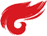KMP算法详解

KMP算法得核心就是对PMT得理解，下面介绍PMT：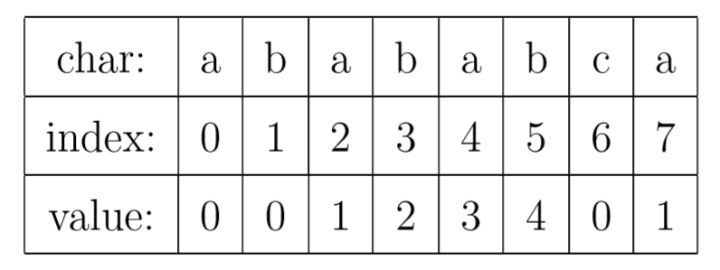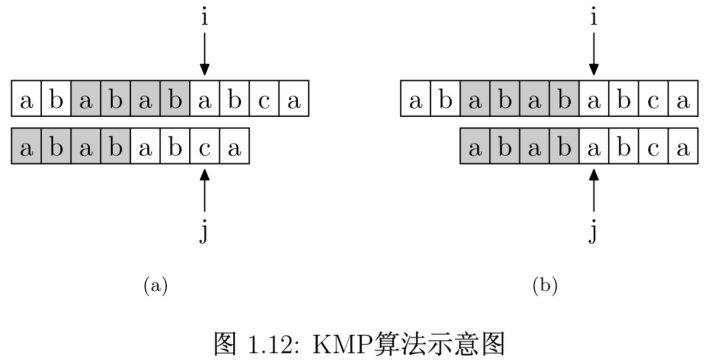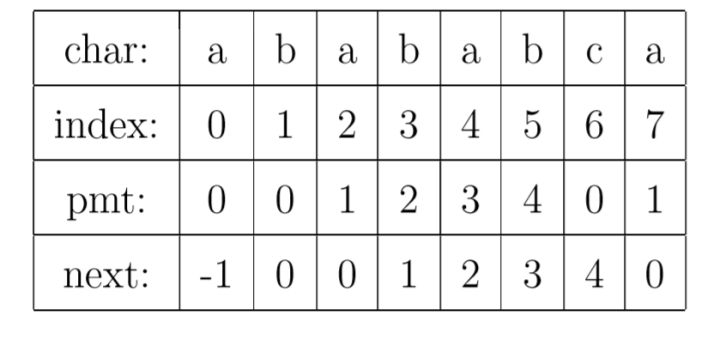1. int KMP(char * t, char * p)
2. {
3. int i = 0;
4. int j = 0;
5.
6. while (i < strlen(t) && j < strlen(p))
7. {
8. if (j == -1 || t[i] == p[j])
9. {
10. i++;
11. j++;
12. }
13. else
14. j = next[j];
15. }
16.
17. if (j == strlen(p))
18. return i - j;
19. else
20. return -1;
21. }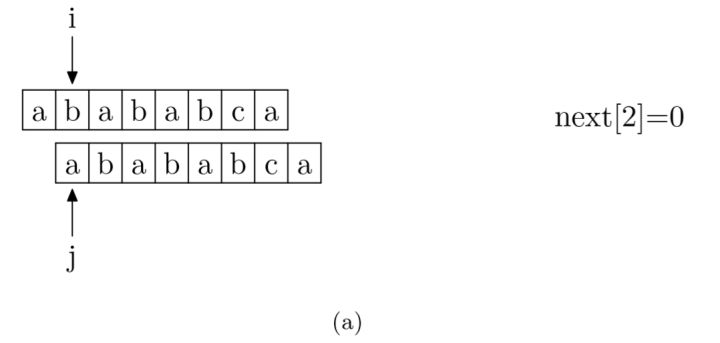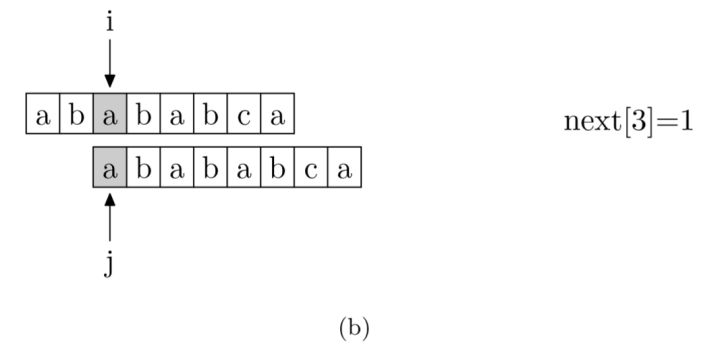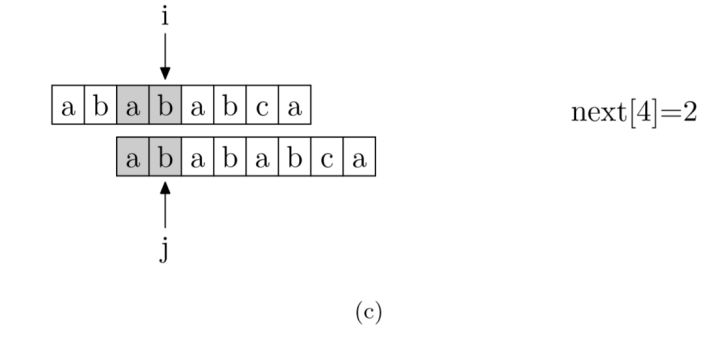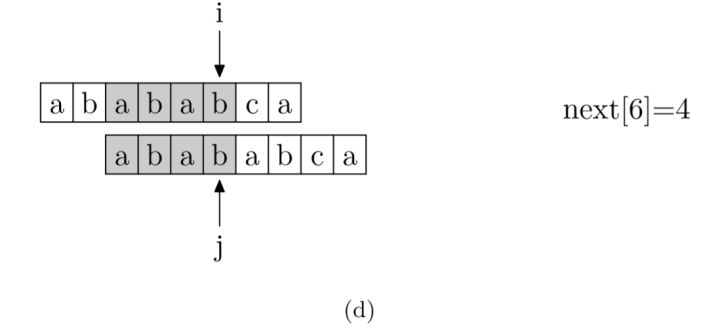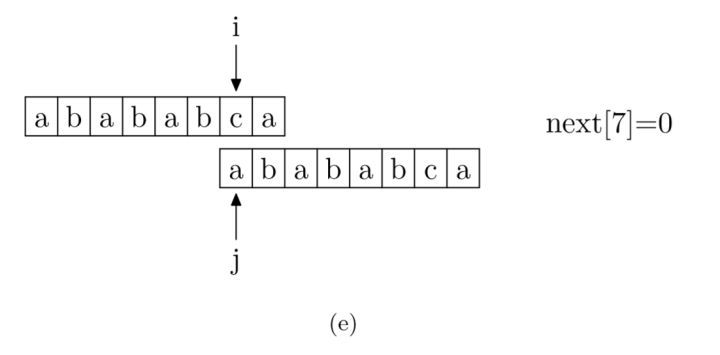1. void getNext(char * p, int * next)
2. {
3. next = -1;
4. int i = 0, j = -1;
5.
6. while (i < strlen(p))
7. {
8. if (j == -1 || p[i] == p[j])
9. {
10. ++i;
11. ++j;
12. next[i] = j;
13. }
14. else
15. j = next[j];
16. }
17. }
posted on 2019-05-17 17:15  幽love梦费重寻  阅读(124)  评论(0编辑  收藏  举报